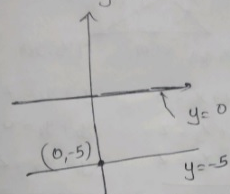Question

# The pair of equations and has

A

B

C

D

## No solutions

Medium
SolutionVerified by Toppr
Correct option is D)

## Explanation:Since, given variable y has different values so, The pair of equations and has no solution.Therefore the correct option is 'D' is No solutions.00
Similar questions